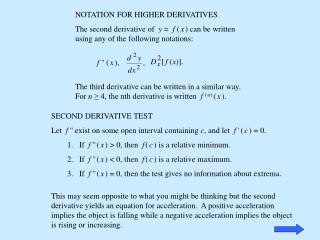Download PresentationNOTATION FOR HIGHER DERIVATIVES

# NOTATION FOR HIGHER DERIVATIVES - PowerPoint PPT PresentationDownload Presentation## NOTATION FOR HIGHER DERIVATIVES

- - - - - - - - - - - - - - - - - - - - - - - - - - - E N D - - - - - - - - - - - - - - - - - - - - - - - - - - -
##### Presentation Transcript

1. NOTATION FOR HIGHER DERIVATIVES The second derivative of y = f (x) can be written using any of the following notations: f'' (x), The third derivative can be written in a similar way. For n≥ 4, the nth derivative is written f(n)(x). • SECOND DERIVATIVE TEST • Let f'' exist on some open interval containing c, and let f '(c) = 0. • If f ''(x) > 0, then f(c) is a relative minimum. • If f ''(x) < 0, then f(c) is a relative maximum. • If f ''(x) = 0, then the test gives no information about extrema. This may seem opposite to what you might be thinking but the second derivative yields an equation for acceleration. A positive acceleration implies the object is falling while a negative acceleration implies the object is rising or increasing.

2. Second Derivative TestProblem 2 2. Find the x-values of all points where the following has any relative maxima or relative minima using the second derivative test. Then find the value of any relative maxima or relative minima writing the answer in order pair form. Step 1. Find the first derivative. Step 2. Set the first derivative equal to zero and solve. (5x 3)(2x + 7) = 0 2x + 7 = 0 2x =  7 x = 5x 3 = 0 5x = 3x =

3. Step 3. Find the second derivative. Step 4. Evaluate the second derivative with each critical point found in step 2. Determine if the critical point is a relative maximum or relative minimum. These numbers are not your answers. Do not say they are the answers. They just determine the type of answers. Step 5. Substitute each c into the original equation.

4. Step 6. Write answers as ordered pairs The – 41 in step 4 indicates the critical point is a relative maximum. The 41 in step 4 indicates the critical point is a relative minimum. Answers: is a relative maximum. is a relative minimum. Pay attention to the order of the animation. I am trying to show the order in which things done and the way the answers are to be written. Just reading this slide from top to bottom does not accomplish that.

5. I do not expect you to sketch the graph on a test.